# Visualizing meta-analytic data with R package metaviz

#### 2020-04-07

The R package metaviz is a collection of functions to create visually appealing and information-rich plots of meta-analytic data using ggplot2. Currently functions to create several variants of forest plots (`viz_forest`) and funnel plots (`viz_funnel`, `viz_sunset`) are provided. See the function documentations for more details and relevant references.

This vignette is a tutorial for the use of metaviz to visualize meta-analytic data and presents some of its main features.

In the following, we use four different example datasets distributed with the package metaviz. The datasets `mozart` and `homeopath` include published meta-analyses using the Cohen d metric (Pietschnig, Voracek, & Formann, 2010; Mathie et al. 2017), `brainvol` contains data for a meta-analysis using correlation coefficients (Pietschnig et al., 2015), and `exrehab` contains data of a published meta-analysis with dichotomous outcome data (Anderson et al. 2016). More details can be found in the respective help files (`help(mozart)`, `help(homeopath)`, `help(brainvol)`, `help(exrehab)`).

The main input of functions in package metaviz is a data.frame or matrix with the study effect sizes in the first column and the respective standard errors in the second column. Alternatively, the output of the function `rma.uni` from R package metafor can be supplied as input, then effect sizes and standard errors are taken from there.

First, the R package metaviz needs to be installed and attached within the R environment.

``library(metaviz)``

## Creating forest plots with function viz_forest

The `viz_forest` function is the main function in metaviz to create forest plots and their variants. Many options to visually customize the forest plot are provided (see `help(viz_forest)`)

``````viz_forest(x = mozart[1:10, c("d", "se")], study_labels = mozart[1:10, c("study_name")],
summary_label = "Summary effect", xlab = "Cohen d")``````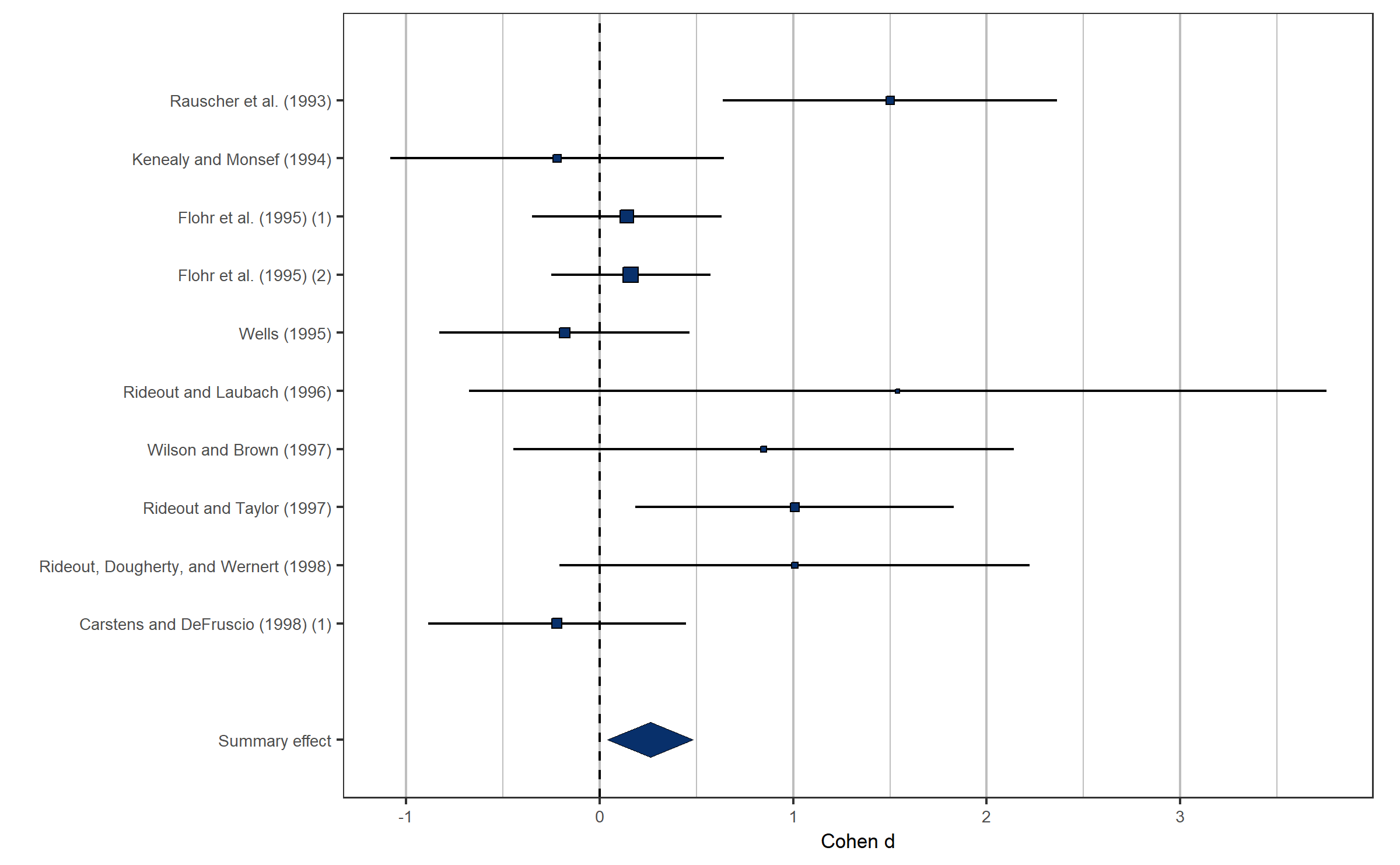### Forest plot variants: rainforest plot and thick forest plots

In addition to traditional forest plots, rainforest plots as well as thick forest plots can be created via the `variant` argument. Alternatively, the wrapper functions `viz_thickforest` and `viz_rainforest` can be used with identical results. Rainforest and thick forest plots are two variants and enhancements of the classical forest plot recently proposed by Schild and Voracek (2015). Both variants visually emphasize large studies (with short confidence intervals and more weight in the meta-analysis), while small studies (with wide confidence intervals and less weight in the meta-analysis) are visually less dominant. For further details see `help(viz_rainforest)` and `help(viz_thickforest)`.

``````viz_forest(x = mozart[1:10, c("d", "se")], study_labels = mozart[1:10, c("study_name")],
summary_label = "Summary effect", xlab = "Cohen d", variant = "rain")``````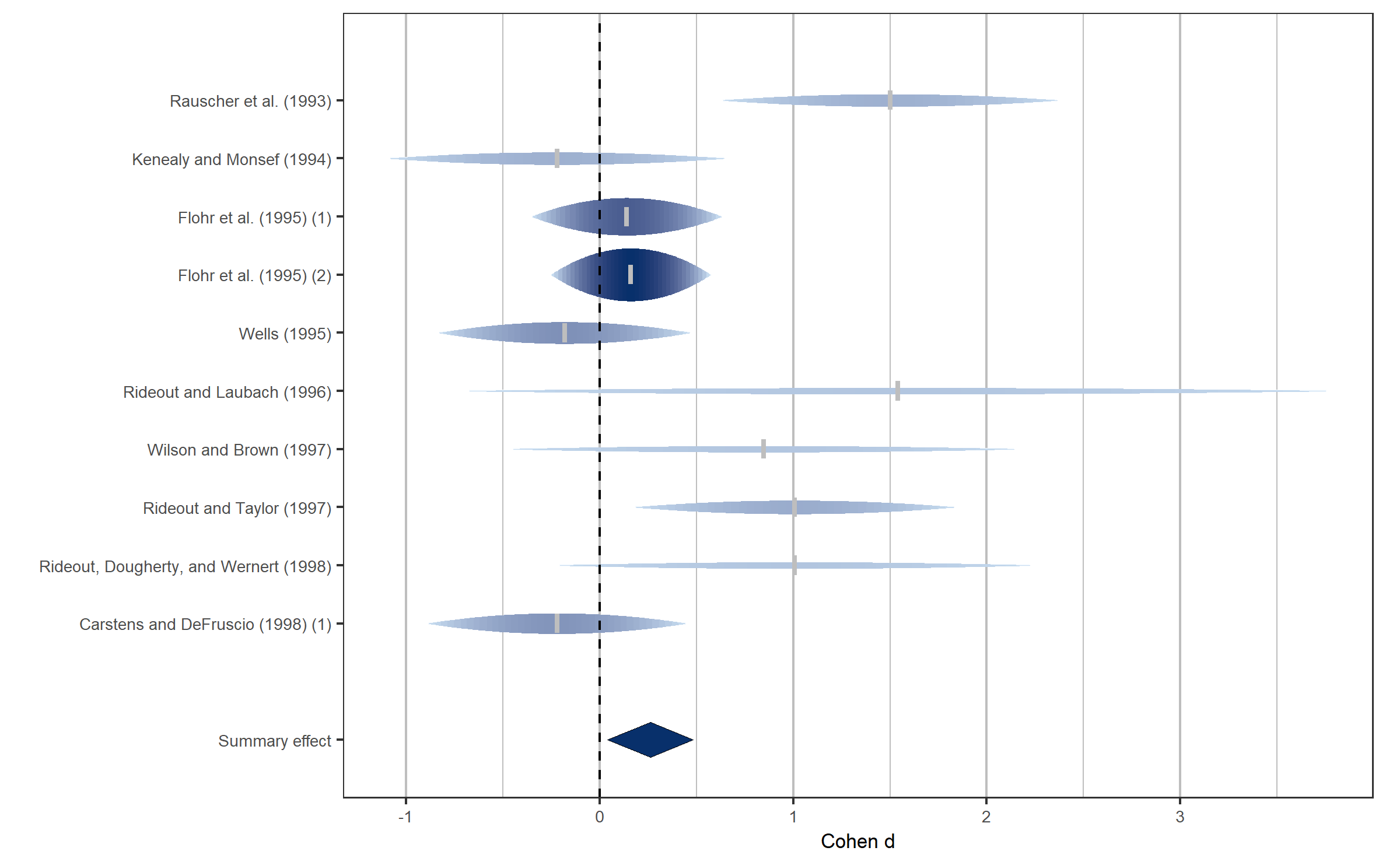``````viz_forest(x = mozart[1:10, c("d", "se")], study_labels = mozart[1:10, c("study_name")],
summary_label = "Summary effect", xlab = "Cohen d", variant = "thick", method = "FE")``````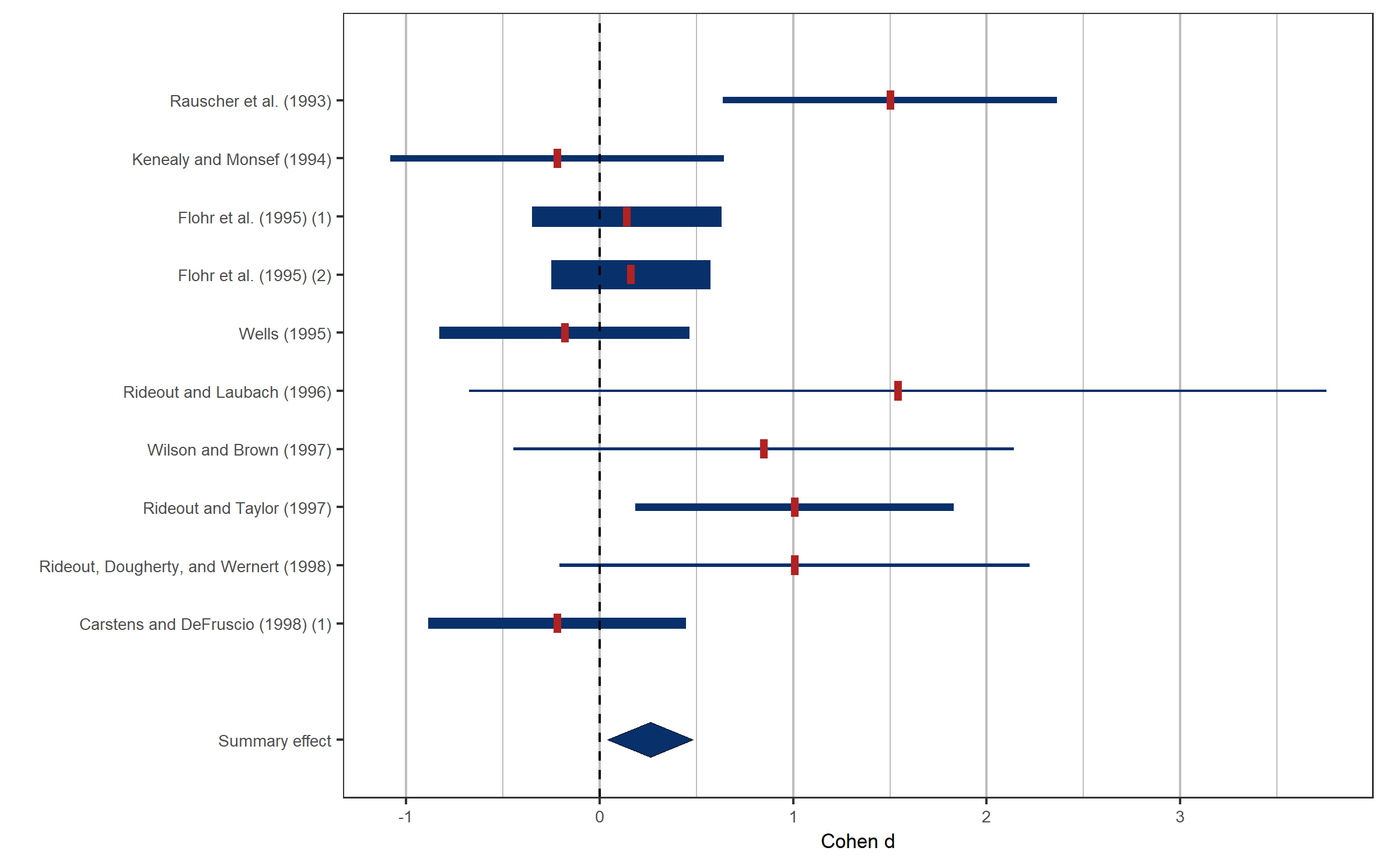The `method` argument controls the meta-analytic model (fixed effect or random effects model). Setting `method` from a fixed effect to a random effects model changes the estimated summary effect and meta-analytic (inverse-variance) weights assigned to each study accordingly.

``````viz_forest(x = mozart[1:10, c("d", "se")], study_labels = mozart[1:10, c("study_name")],
summary_label = "Summary effect", xlab = "Cohen d", variant = "rain", method = "DL")``````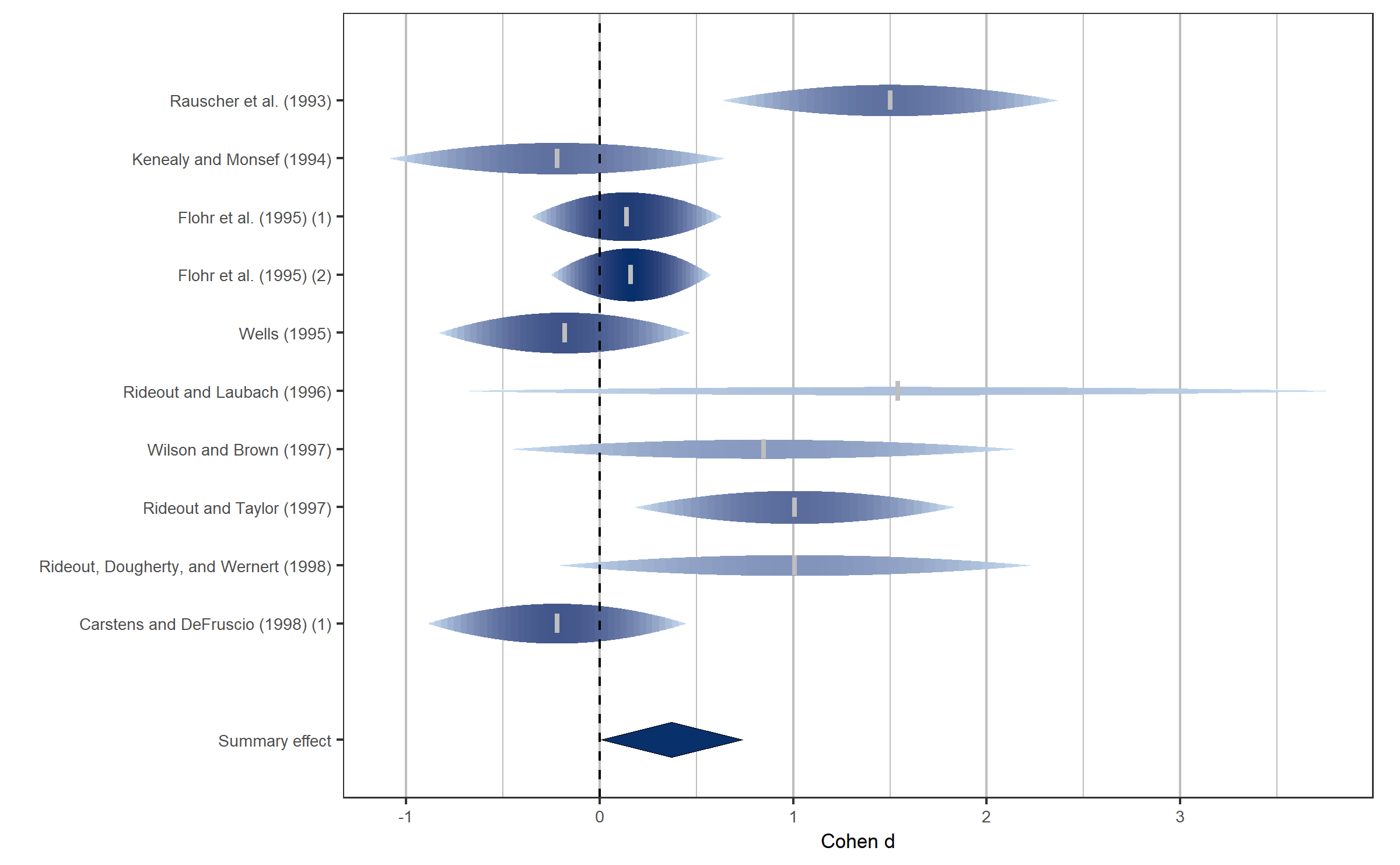### Subgroup analysis

The `viz_forest` function is able to use a categorical moderator variable to visualize a subgroup analysis. This is done via the `group` argument, a factor which corresponds to the subgroup membership of each study. We use the dichotomous moderator `rr_lab` to compute and visualize separate meta-analyses. In the case of subgroup analysis, the `summary_label` argument can be a vector containing names for all subgroups, arranged in the order of the levels of `group`.

``````viz_forest(x = mozart[1:10, c("d", "se")],
group = mozart[1:10, "rr_lab"],
study_labels = mozart[1:10, "study_name"],
summary_label = c("Summary (rr_lab = no)", "Summary (rr_lab = yes)"),
xlab = "Cohen d",
col = "Greys",
variant = "rain")``````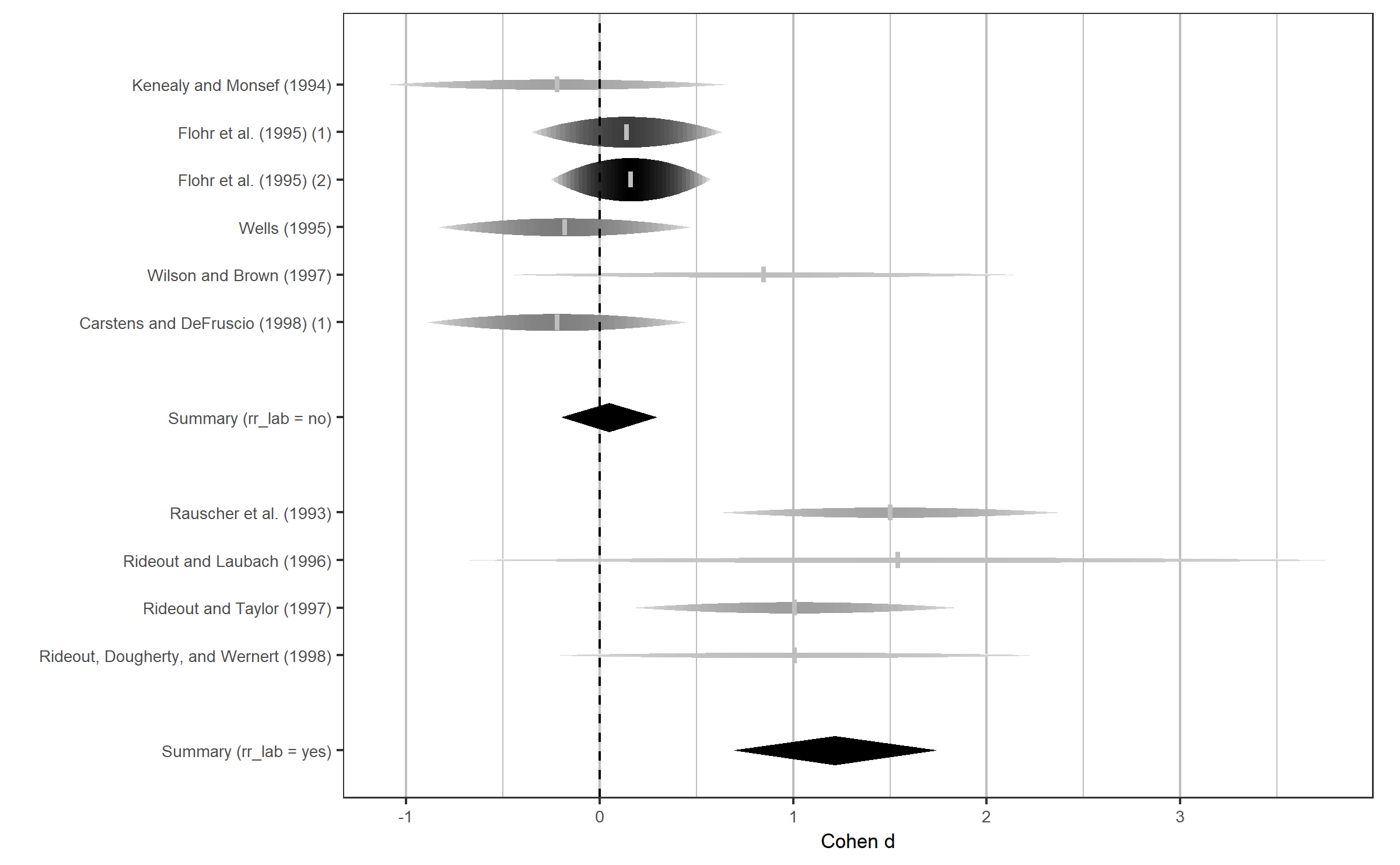### Available forest plots types: Cumulative and leave-one-out analysis.

Different aspects of meta-analytic data can be shown in forest plots. Within function `viz_forest` the `type` parameter controls which aspects are shown. Two examples are given below.

Argument `type = "cumulative"` shows a cumulative meta-analysis, that is, meta-analytic summary effects are computed sequentially by adding each study one-by-one.

``````viz_forest(x = mozart[, c("d", "se")],
group = mozart[, "rr_lab"],
study_labels = mozart[, "study_name"],
summary_label = c("Summary (rr_lab = no)", "Summary (rr_lab = yes)"),
xlab = "Cohen d",
variant = "thick",
type = "cumulative")``````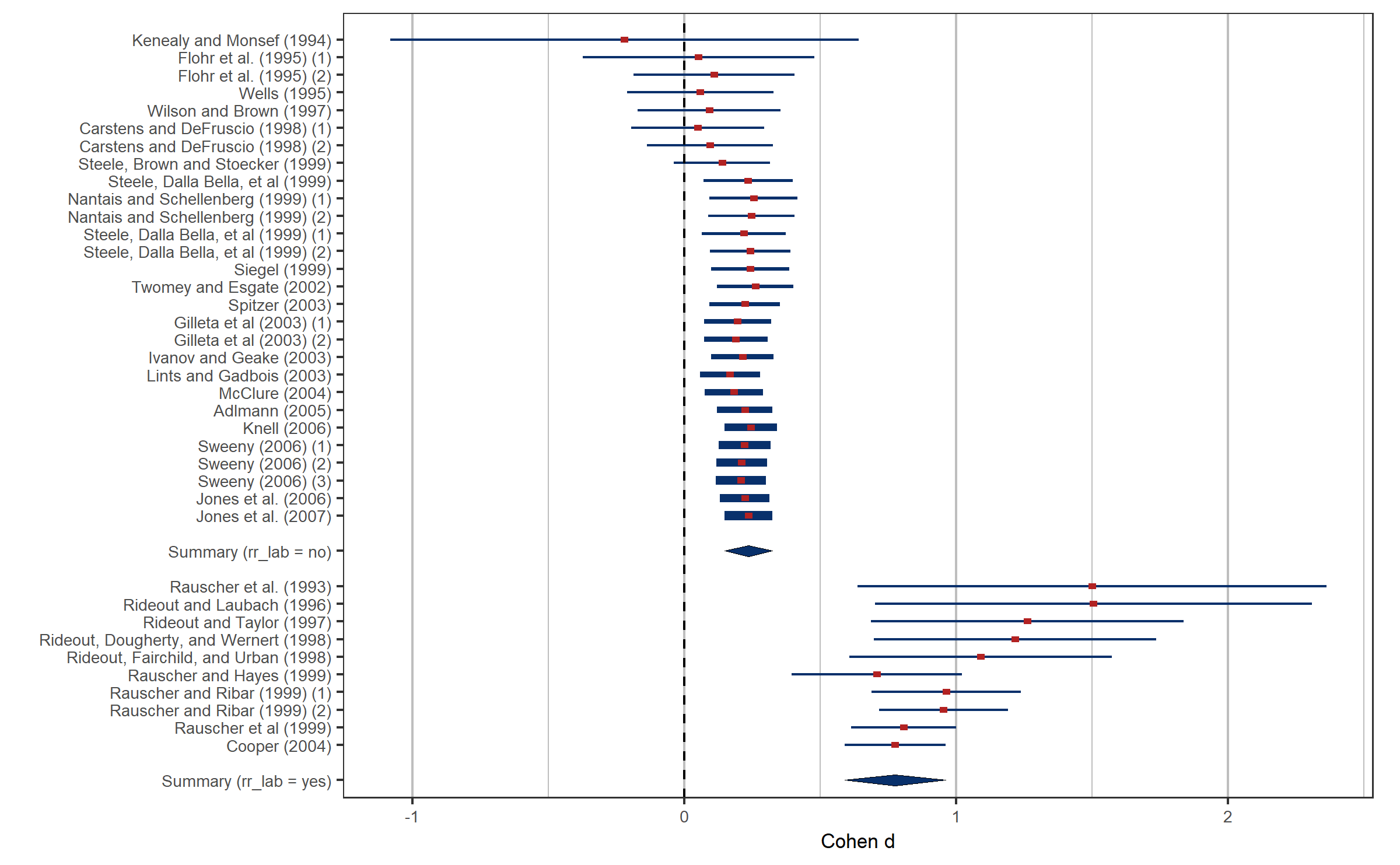Argument `type = "sensitivity"` shows a leave-one-out analysis. That is, for each study the meta-analytic summary effect is shown if that particular study is not considered in the computation of the summary effect.

``````viz_forest(x = mozart[1:5, c("d", "se")],
study_labels = mozart[1:5, "study_name"],
xlab = "Cohen d",
variant = "rain",
type = "sensitivity")``````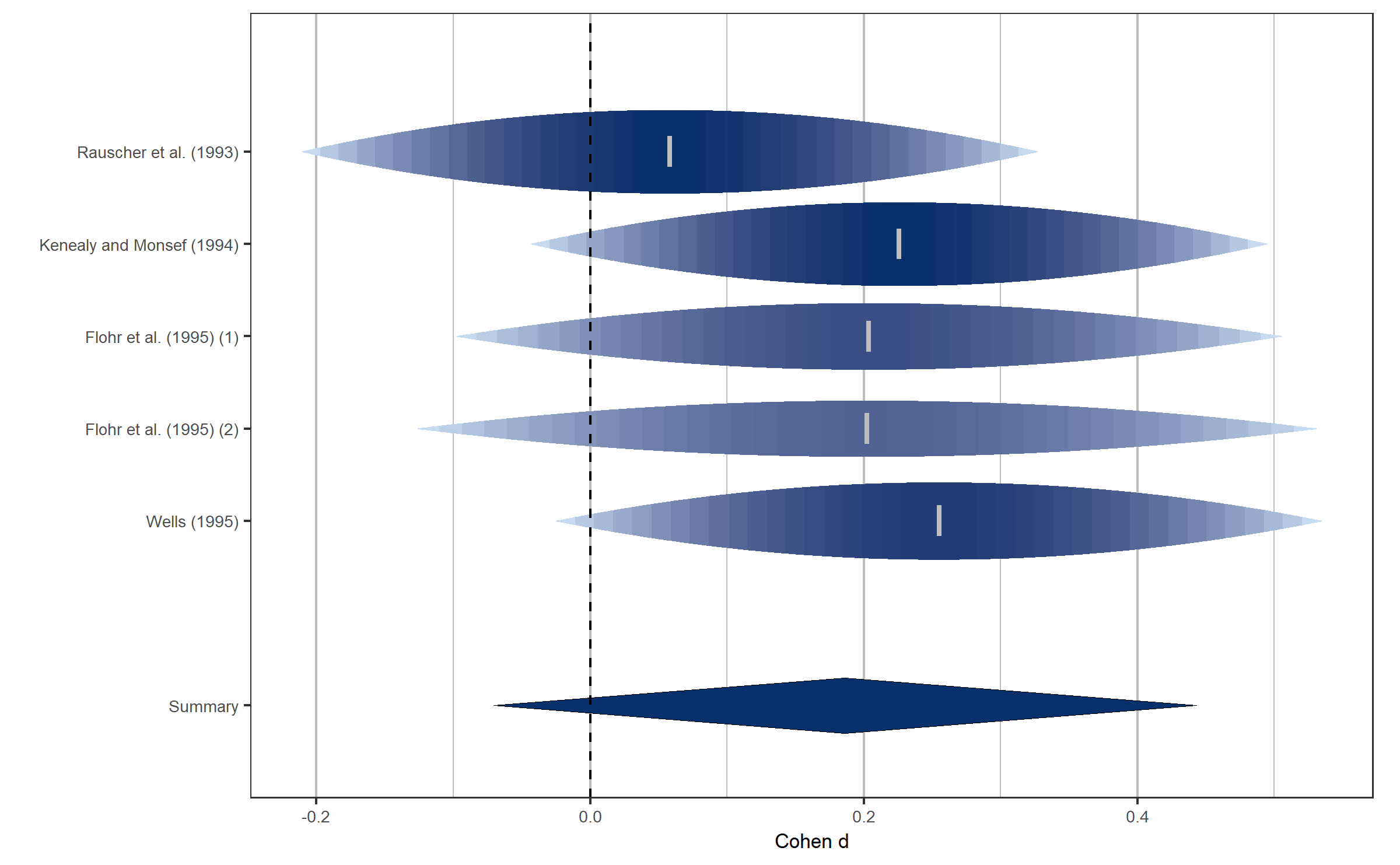### Aligning tables with study and/or summary information

The `viz_forest` function was developed to allow aligning a table to the forest plot containing study or summary information. First, textual annotations of effect sizes and confidence intervals can be optionally displayed with the argument `annotate_CI = TRUE`.

``````viz_forest(x = mozart[1:10, c("d", "se")],
group = mozart[1:10, "rr_lab"],
study_labels = mozart[1:10, "study_name"],
summary_label = c("Summary (rr_lab = no)", "Summary (rr_lab = yes)"),
xlab = "Cohen d",
variant = "thick",
annotate_CI = TRUE)``````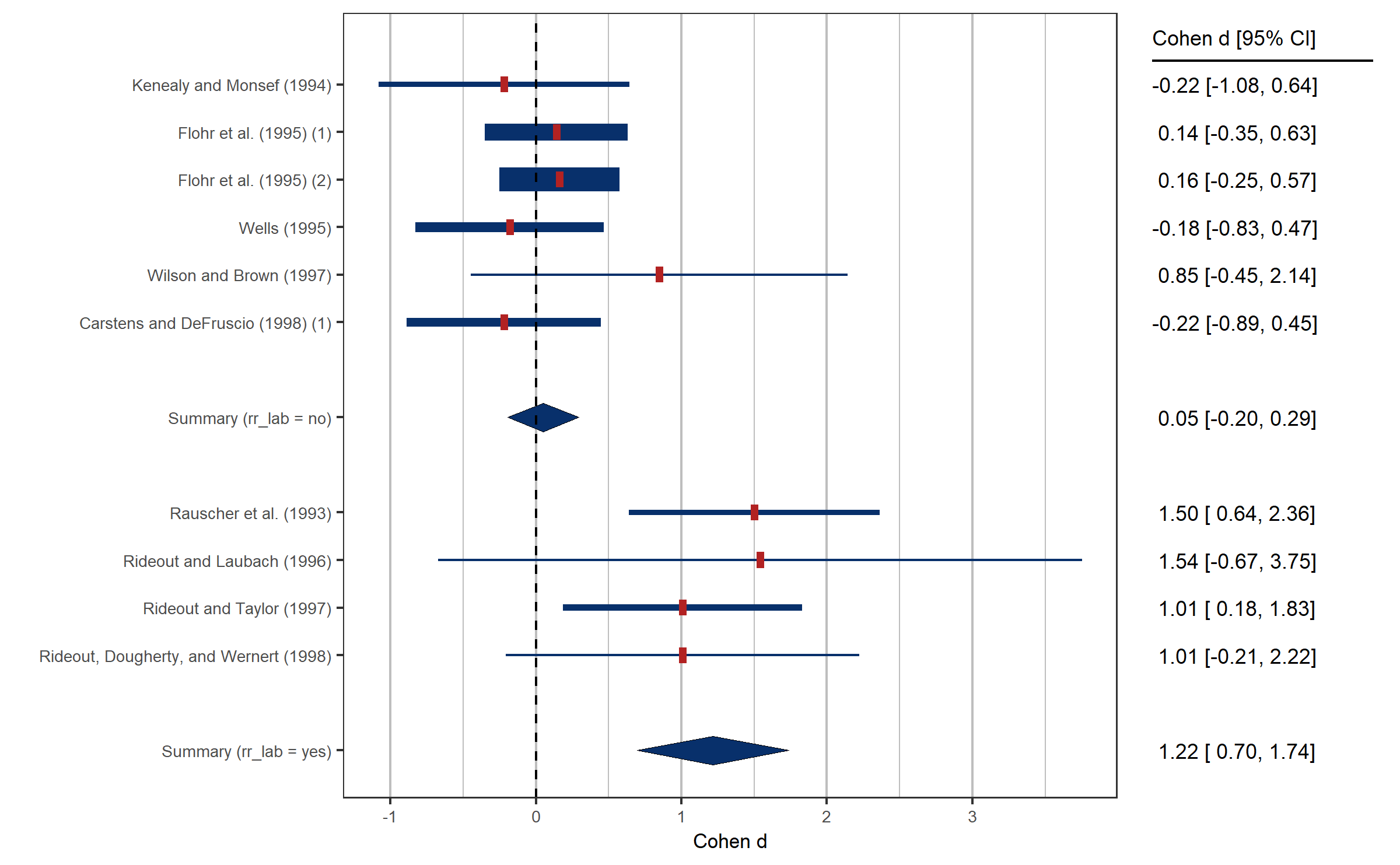Second, arbitrary study information can be supplied as data.frame with the argument `study_table`. This data.frame can contain several variables to be aligned as columns and should contain one row for each study in the meta-analysis.

To illustrate, we might want to align a table with the study identifiers and number of events observed in each study of data set `exrehab`.

``````study_table <- data.frame(
name = exrehab[, "study_name"],
eventsT = paste(exrehab\$ai, "/", exrehab\$ai + exrehab\$bi, sep = ""),
eventsC = paste(exrehab\$ci, "/", exrehab\$ci + exrehab\$di, sep = ""))
``````##              name eventsT eventsC
## 1 Shaw (NEDHP) 81 109/323 113/328
## 2        Lewin 92    9/58   18/58
## 3      Haskell 94  62/145  72/155
## 4       Englom 96  26/102   34/91
## 5  Hofman-Bang 99   19/46   21/41
## 6 Belardinelli 01   11/59   21/59``````

We might also want to include the sum of all events as summary information. This can be done with the `summary_table` argument. `summary_table` should be a data.frame with the number of rows equal to the number of subgroups.

``````summary_table <- data.frame(
name = "Summary",
eventsT = paste(sum(exrehab\$ai), "/", sum(exrehab\$ai + exrehab\$bi), sep = ""),
eventsC = paste(sum(exrehab\$ci), "/", sum(exrehab\$ci + exrehab\$di), sep = ""))
``````##      name  eventsT  eventsC
## 1 Summary 407/1471 453/1388``````

The table_headers of `study_table` and `summary_table` can be specified with `table_headers`.

``````viz_forest(x = exrehab[, c("logrr", "logrr_se")], variant = "classic",
col = "Greys", xlab = "logRR", annotate_CI = T,
study_table = study_table,
summary_table = summary_table,
table_headers = c("ID", "Events (T)", "Events (C)"))``````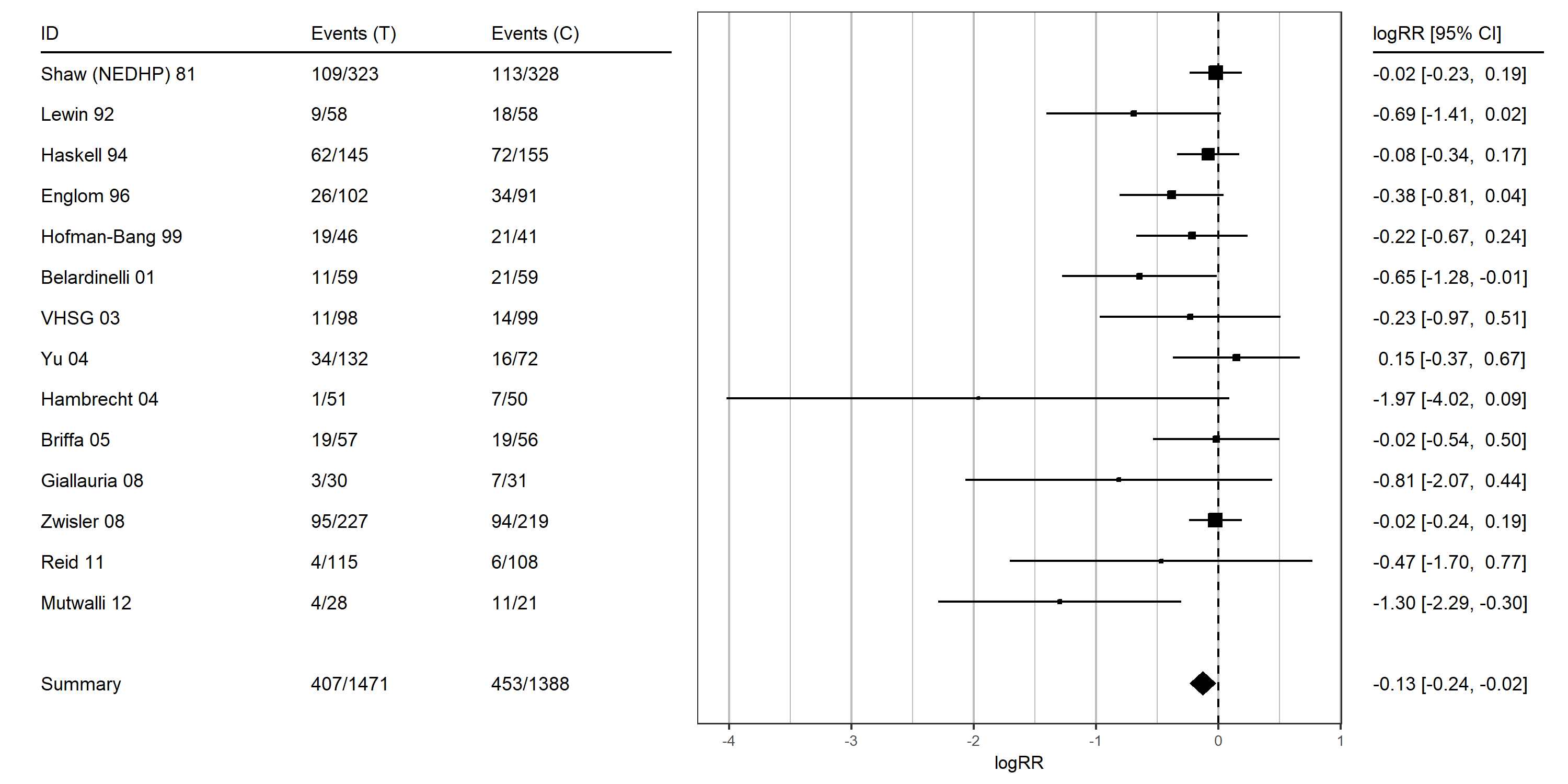The spacing of the forest plot and aligned tables can be customized by supplying a layout matrix via `table_layout`.

``````viz_forest(x = exrehab[, c("logrr", "logrr_se")], variant = "classic",
col = "Greys", xlab = "logRR", x_limit = c(-0.35, 0.05),
annotate_CI = T, type = "sensitivity",
study_table = data.frame(left_out = exrehab[, "study_name"],
remaining_N = sum(exrehab[, "n1i"] + exrehab[, "n2i"]) -
(exrehab[, "n1i"] + exrehab[, "n2i"])),
summary_table = "None",
table_headers = c("Study left out", "N remaining", "log Risk Ratio [95% CI]"),
table_layout = matrix(c(1, 2, 3), nrow = 1))``````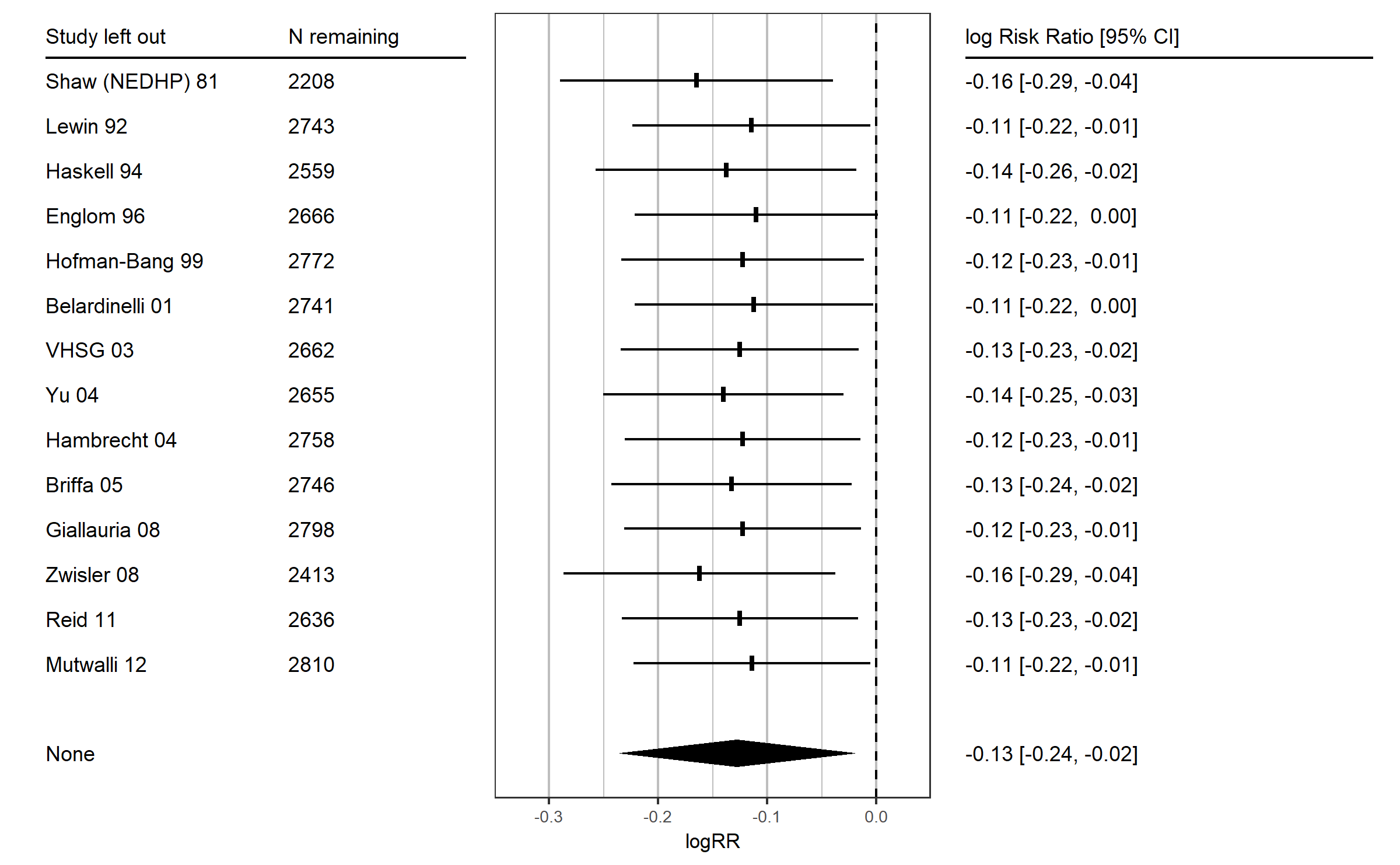### Transforming the x axis labels

For some transformed effect sizes (e.g., log odds ratios, log risk ratios, or Fisher’s z) it is good practice to transform the labels of the x-axis, such that they display the effect sizes on their original scale (e.g., odds, ratios, risk ratios, or correlations). This can be conveniently done with the argument `x_trans_function`.

``````viz_forest(x = exrehab[, c("logrr", "logrr_se")], variant = "thick",
study_labels = exrehab[, "study_name"], col = "Greys",
study_table = study_table,
summary_table = summary_table,
annotate_CI = T,
table_headers = c("ID", "Events (T)", "Events (C)"),
x_trans_function = exp, xlab = "RR")``````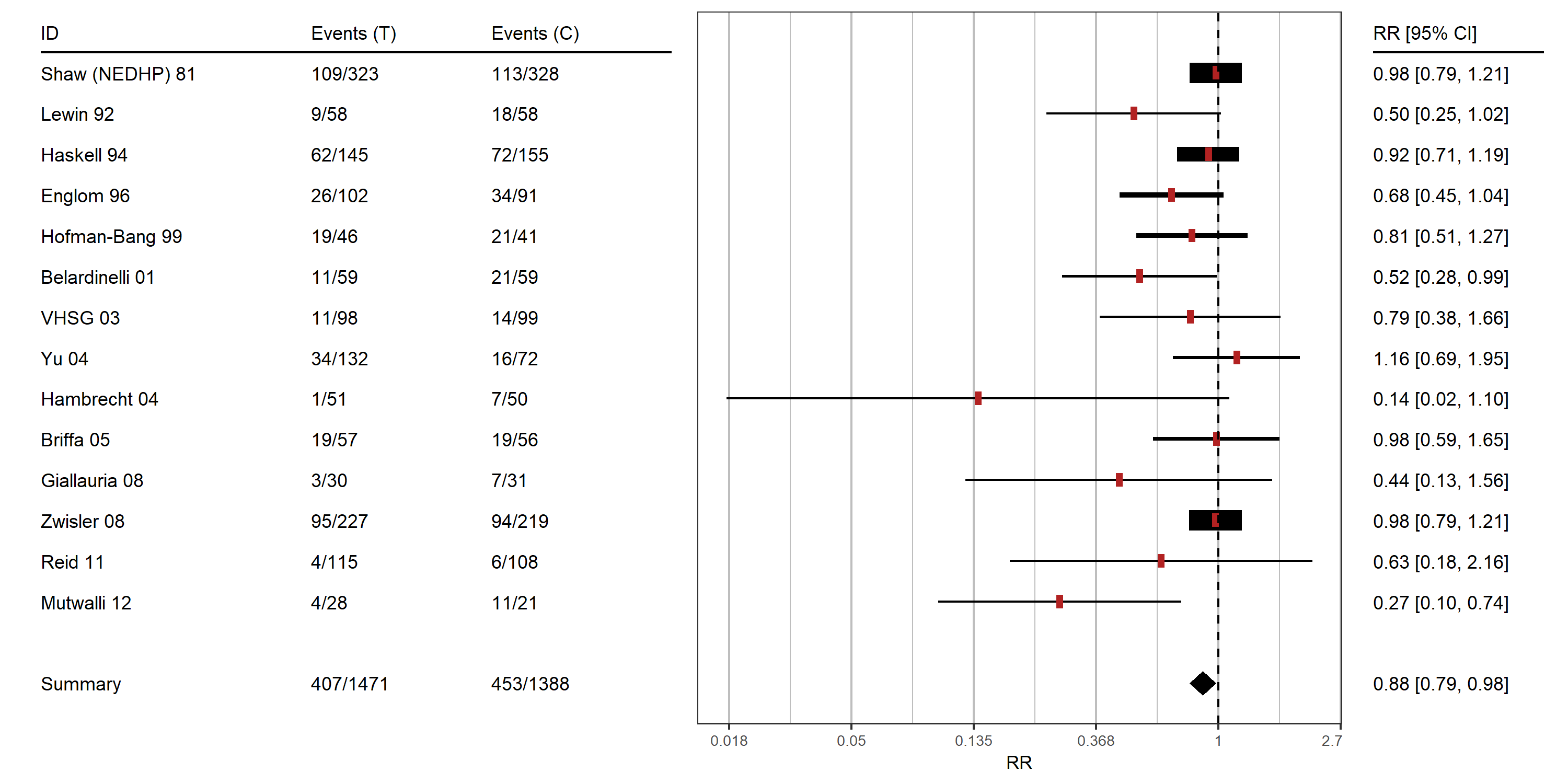### Color highlighting single studies or groups of studies

For forest plots and thick forest plots it is possible to individually customize the color of studies by supplying a vector of colors to the `col` argument. The color of the summary effect(s) can be customized individually as well with the `summary_col` argument.

``````viz_forest(x = mozart[1:10, c("d", "se")],
group = mozart[1:10, "rr_lab"],
study_labels = mozart[1:10, "study_name"],
summary_label = c("Summary (rr_lab = no)", "Summary (rr_lab = yes)"),
xlab = "Cohen d",
col = c("firebrick", "steelblue4")[mozart[1:10, "rr_lab"]],
summary_col = c("firebrick", "steelblue4"))``````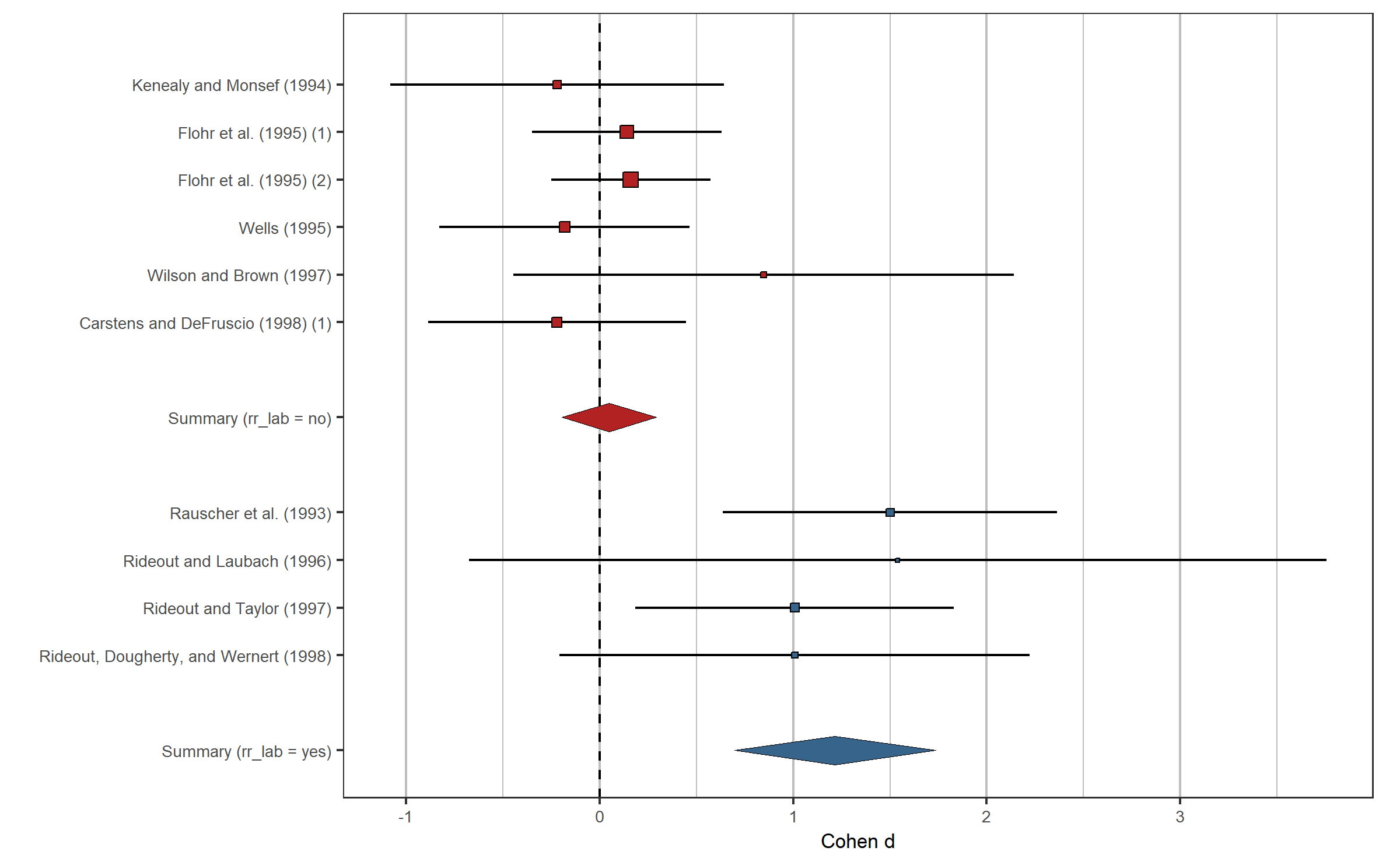## Creating funnel plots with viz_funnel

The function `viz_funnel` is capable to create a large set of different funnel plot variants. Options for several graphical augmentations (e.g., confidence, significance, and additional evidence contours; choice of the ordinate; showing study subgroups), and different statistical information displayed are provided (Egger’s regression line, and imputed studies by, as well as the adjusted summary effect from, the trim-and-fill method). See `help(viz_funnel)` for further details and relevant references.

By default, significance contours (for the 5% and 1% level) and 95% confidence contours (fixed effect model) are shown.

``viz_funnel(brainvol[, c("z", "z_se")])``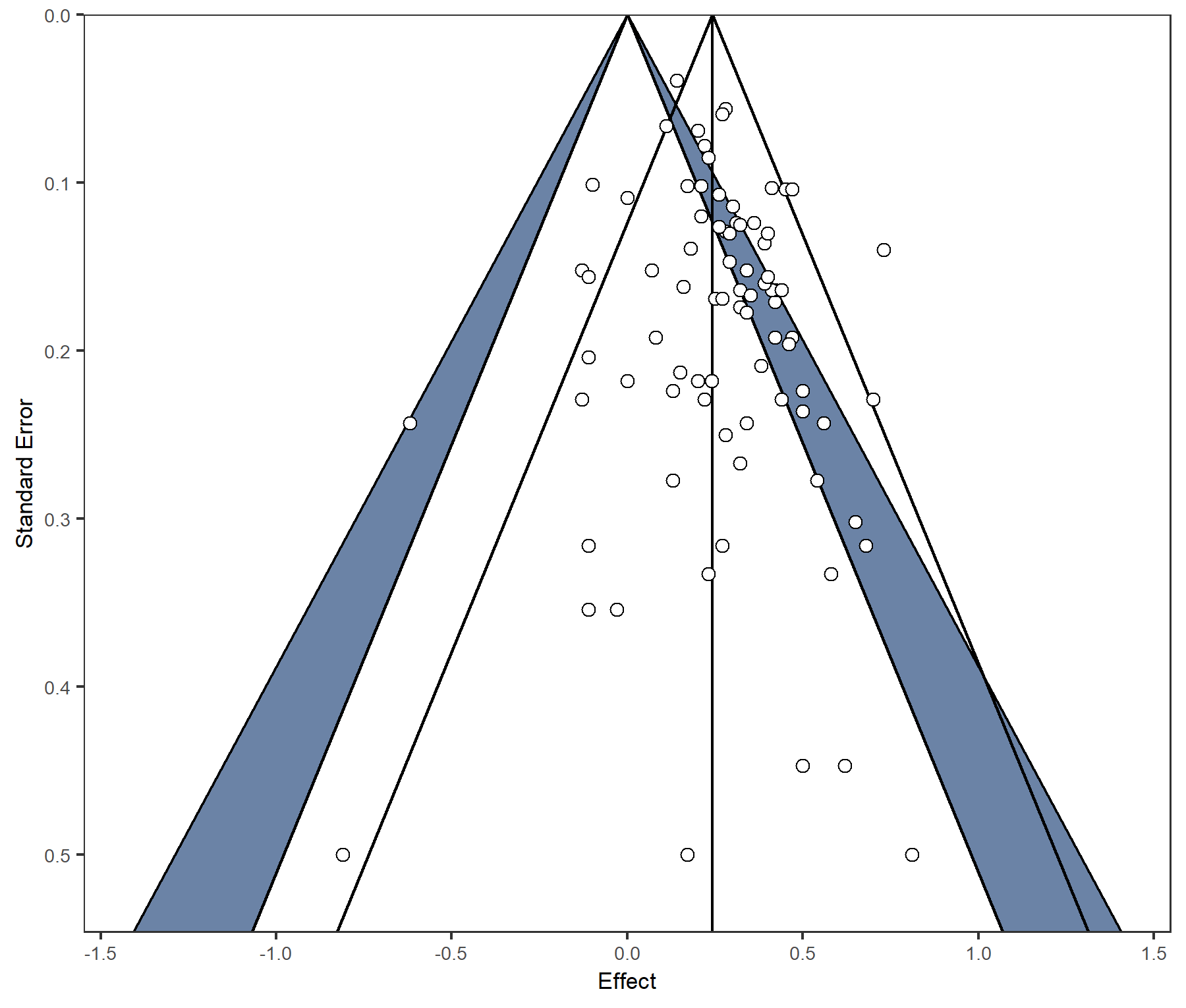In the `brainvol` meta-analysis Fisher’s z values are used. It is convenient to show Fisher’s z values on their original scale (correlations) using the `x_trans_function`. The meta-analytic model can be changed to a random effects model using `method`. Note that for a random effects meta-analysis, confidence contours do not necessarily converge to a single point for a standard error of zero.

``````viz_funnel(brainvol[, c("z", "z_se")],
method = "DL",
contours_col = "Greys",
xlab = "r", x_trans_function = tanh,
x_breaks = atanh(c(-0.9, -0.7, -0.3, 0, 0.3, 0.7, 0.9)))``````